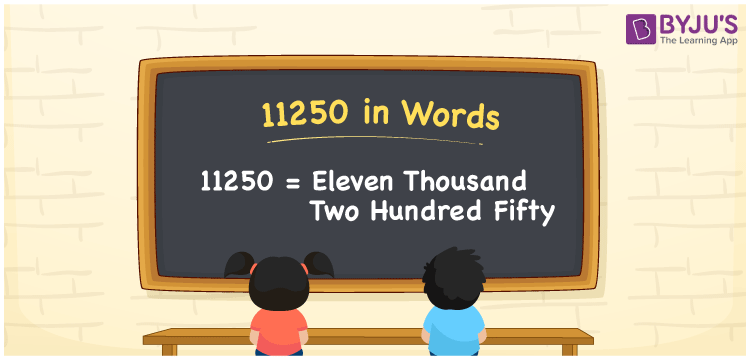# 11250 in Words

11250 in words is “Eleven thousand two hundred fifty”. As we all know, 11250 is a cardinal number that denotes the object’s value or count. The place value table can be used to simply convert the number 11250 into words. For example, we can write “The cost of the bench is Rs. Eleven thousand two hundred fifty” instead of “The cost of the bench is Rs. 11250”. Here, We will discuss how to write the number 11250 in words, along with a detailed explanation.

 11250 in Words: Eleven Thousand Two Hundred Fifty. Eleven Thousand Two Hundred Fifty in Numerical Form: 11250.

## 11250 in English Words

In English words, the number 11250 can be written as follows:## How to Write 11250 in Words?

The number 11250 can be written in words using the place value system. The place value table for the number 11250 is shown below.

 Ten-thousands Thousands Hundreds Tens Ones 1 1 2 5 0

The following is the expanded form of the number 11250:

= 1 × Ten thousand + 1 × Thousand + 2 × Hundred + 5 × Ten + 0 × One

= 1 × 10000 + 1 × 1000 + 2 × 100 + 5 × 10

= 10000 + 1000 + 200 + 50

= 11250

= Eleven thousand two hundred fifty

Hence, 11250 in words is eleven thousand two hundred fifty.

The following are the facts about the number 11250.

11250 in words – Eleven thousand two hundred fifty

Is 11250 an odd number? – No

Is 11250 an even number? – Yes

Is 11250 a perfect square number? – No

Is 11250 a perfect cube number? – No

Is 11250 a prime number? – No

Is 11250 a composite number? – Yes

## Frequently Asked Questions on 11250 in Words

Q1

### How to write 11250 in words?

11250 in words can be written as eleven thousand two hundred fifty.

Q2

### Simplify 11300 – 50, and express in words.

Simplifying 11300 – 50, we get 11250. Hence, 11250 in words is eleven thousand two hundred fifty.

Q3

### Convert eleven thousand two hundred fifty into numbers.

Eleven thousand two hundred fifty in numerals is 11250.

Test your Knowledge on 11250 in Words